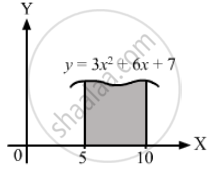Department of Pre-University Education, KarnatakaPUC Karnataka Science Class 11

# Find the Area Bounded Under the Curve Y = 3x2 + 6x + 7 and the X-axis with the Ordinates at X = 5 and X = 10. - Physics

Sum

Find the area bounded under the curve y = 3x2 + 6x + 7 and the X-axis with the ordinates at x = 5 and x = 10.

#### Solution

The given equation of the curve is y = 3x2 + 6x + 7.The area bounded by the curve and the X-axis with coordinates x1 = 5 and x2 = 10 is given by

$\int_{x_1}^{x_2} y dx$

$= \int_5^{10} \left( 3 x^2 + 6x + 7 \right)dx$

$= \left[ \frac{3 x^3}{3} + \frac{6 x^2}{2} + 7x \right]_5^{10}$

$= 1000 - 125 + 300 - 75 + 70 - 35$

$= 1370 - 235$

= 1135 sq. units

Concept: Physics Related to Technology and Society
Is there an error in this question or solution?

#### APPEARS IN

HC Verma Class 11, Class 12 Concepts of Physics Vol. 1
Chapter 2 Physics and Mathematics
Exercise | Q 24 | Page 29

Share